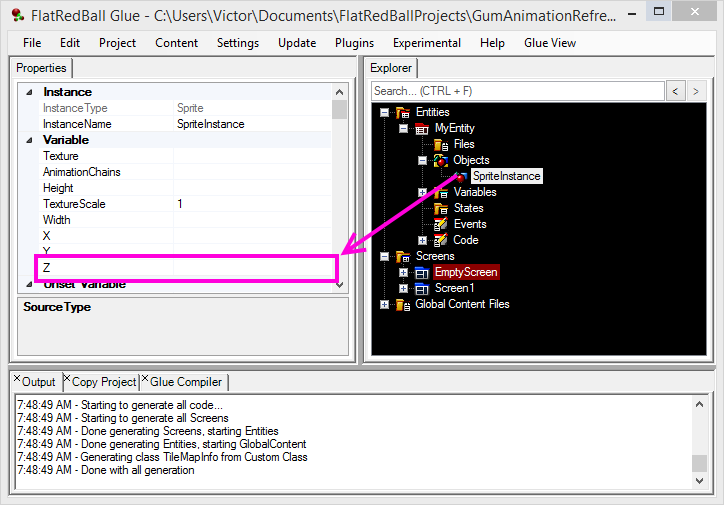# Z

## Introduction

The Z value of an entity controls its distance away from the camera. If using a 3D Camera/Layer then making the Z a smaller (including negative) number will make the entity smaller and will make it sort behind other graphical objects. If using a 2D Camera/Layer then the Z value will not change the Entity’s size, but will impact its sorting.

The Z property is a property which comes from the PositionedObject class. More information on the Z value can be found here.

You can change the Z of most objects in Glue through the property grid:## Z and Ordering

The Z value of an object also controls the order that it is drawn. An object with a Z value of 0 will be drawn under an object with a Z value of 1. In other words, objects with a smaller Z will be drawn under objects with a larger Z.

### Important: Objects with the same Z will drawn in an undefined order

If two objects overlap and have the same Z, then the order in which they are drawn is undefined. Therefore, if you intend on having one object drawn on top of another, you should explicitly set the Z values of the objects. If you have two objects which overlap and one is not drawing, you may want to check the Z values to see if this ist he cause of the drawing problem.

## Z may be absolute or relative

The Z value in Glue is either absolute or relative depending on when the value is set and what the object/Entity is attached to. For example, setting the Z value of an Entity which is not attached to anything else in a Screen will set its absolute Z value. However, setting the Z value on an object inside of an Entity will actually set the RelativeZ value – the actual Z value depends on where the container Entity is positioned.

For example, consider an Entity called Player which has a Circle object. In this situation the Circle’s Z is set to 1. This means that the Circle’s Z will be 1 + the Entity’s Z. Therefore, if a Player instance were placed in a Screen and given a Z value of 3, then the Circle’s absolute Z value would be 3 + 1 = 4.

## Z and AttachToCamera

The AttachToCamera property changes how Z works. An object which has its AttachToCamera set to true and a Z value of 0 will actually have its RelativeZ value set to -40 instead of 0. The reason for this is because it makes things “just work” more often.

If an Entity has a Z value of 0 and it is attached to the Camera, many users expect that the object appears the same as if it were not attached to the Camera. However, technically an object which is attached to the Camera and has a RelativeZ value of 0 would be positioned at the same point as the Camera itself – making it too close to be drawn by the Camera.

Therefore, Glue’s generated code subtracts 40 from the RelativeZ value if the Object’s AttachToCamera is set to true so that default values work without any modifications. In other words, if your Z value is 0, then the RelativeZ on the object at runtime would actually be -40. If your Z value is 10, then the RelativeZ value on the object at runtime would be 10 – 40 = -30

This behavior is rarely something that you need to worry about it, but it is mentioned here in the wiki in case these numbers cause confusion.